# Smart Pointers in C++

## Smart Pointers in C++ with Examples:

In this article, I am going to discuss Smart Pointers in C++ with Examples. Please read our previous article where we discussed Lambda Expressions in C++ with Examples. The Smart Pointers are Introduced in C++ 11.

##### What are Smart Pointers in C++?

Pointers are used for accessing the resources which are external to the program like heap memory. If you are creating anything inside the heap memory then for accessing the heap memory, we need to use pointers. The problem with the heap memory is that when you don’t need it then you must deallocate the memory. And mostly the programmer shows laziness in writing the code for the deallocation of objects from heap memory which causes severe problems like memory leaks which will cause the program to crash.

The languages like JAVA and C# provide a garbage collection mechanism to deallocate the objects from heap memory that is not in use. In C++ 11, smart pointers are introduced which will automatically manage the heap memory and deallocate the object from the heap memory when they are not in use or when the pointer is going out of scope, automatically it will deallocate the memory. So first, I will show you the problem with the normal pointer and then we will see the smart pointers available in C++. Please have a look at the following code.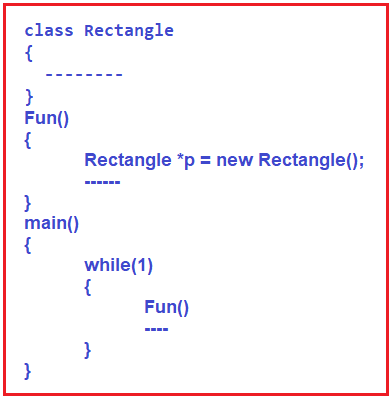Please observe the above. We have Rectangle class, Fun function, and main function. Inside the Main function, we have an infinite while loop. And inside the while loop, we are calling function Fun infinite times. Function fun has a pointer of type Rectangle class. It is dynamically created an object of class Rectangle in the heap memory. For a better understanding, please have a look at the following diagram. Let’s assume that the Rectangle class has two variables called length and breadth of integer types and assume that integer takes 2 Bytes, so for each object creation, it will allocate 4 bytes of memory inside the heap. But the pointer variable p is going to be created inside the stack memory which will point to the heap memory where the actual object is created.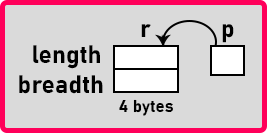Now, once the Fun function execution completes, the p variable will be deleted from the stack memory because it is a local variable to the function Fun but the new Rectangle() that is allocated inside the heap memory will not be deallocated. Then control returns to the main function, and as we have written infinite while loop then again Fun function will be called and another new Rectangle() object will be created inside the heap memory. Pointer p will be deleted when the function ends but these Rectangle objects will not be deleted from the memory. And every time we call the Fun function, it creates the objects but does not delete them from the heap memory. The complete example code is given below.

```#include <iostream>
using namespace std;
class Rectangle
{
int Length;

public:
Rectangle(int l, int b)
{
Length = l;
}
int Area()
{
}
};

int Fun(int l, int b)
{
Rectangle *p = new Rectangle(l, b);
int area = p->Area();
return area;
}

int main()
{
while (1)
{
int Result = Fun(10, 20);
cout << Result << endl;
}
}
```

This will cause leakage of memory from heap memory. Because while the loop is infinite, at one stage because of a lack of heap memory the program will crash. Then what is good practice? At the end of the function fun, we should say delete p as shown in the below image.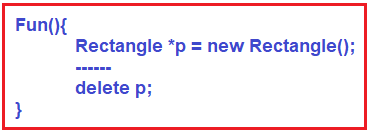The complete example code is given below.

```#include <iostream>
using namespace std;
class Rectangle
{
int Length;

public:
Rectangle(int l, int b)
{
Length = l;
}
int Area()
{
}
};

int Fun(int l, int b)
{
Rectangle *p = new Rectangle(l, b);
int area = p->Area();
delete p;
return area;
}

int main()
{
while (1)
{
int Result = Fun(10, 20);
cout << Result << endl;
}
}
```

Because of the laziness or carelessness of the programmer, this type of problem may arise in the program. Some testing tools also check whether there is a problem of memory leak in the program. But still, it is a good practice to deallocate the unused objects in the program. We can also overcome this problem using Smart Pointers in C++ which was introduced as part of C++ 11.

##### Using Smart Pointers in C++:

If we declare the smart pointer then they will automatically deallocate the object when the smart pointer is going out of the scope. Let us show you how we can declare smart pointers in C++.

##### Unique Pointer in C++: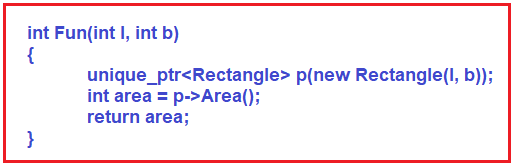Here, inside the Fun function, we have pointer p of type unique_ptr of type Rectangle class. And we have passed the new Rectangle (l, b) as the parameter which will point p to the object of class Rectangle. By using this pointer, we have accessed the Area function of the Rectangle object. In order to use the unique_ptr, we need to include the #include<memory> header file.

So, when the function ends and it is going out of the scope and then the unique pointer p will be deleted and automatically it will also delete the Rectangle object from the heap memory. So, unique_ptr will take care of the deletion of the object from the heap memory. Internally, there is some other pointer that is pointing on the Rectangle object but p is just a variable of type unique_ptr. So now we don’t have to worry about memory leak problems. The complete example code is given below.

```#include <iostream>
#include<memory>
using namespace std;
class Rectangle
{
int Length;

public:
Rectangle(int l, int b)
{
Length = l;
}
int Area()
{
}
};

int Fun(int l, int b)
{
unique_ptr<Rectangle> p(new Rectangle(l, b));
int area = p->Area();
return area;
}

int main()
{
while (1)
{
int Result = Fun(10, 20);
cout << Result << endl;
}
}
```

This is the benefit of using Smart Pointers in C++. Now let us see the other types of smart pointers available in C++ and differentiate between them.

##### unique_ptr:

If you are using unique_ptr, if an object is created and a pointer is pointing to that object then only one pointer can point to that object. So, we cannot share this object with another pointer. But we can transfer the control from one pointer to another pointer by removing p1. So unique_ptr means upon an object at a time only one pointer will be pointing.

##### Example to Understand unique_ptr in C++:
```#include<iostream>
#include<memory>
using namespace std;

class Rectangle
{
int Length;

public:
Rectangle(int l, int b)
{
Length = l;
}
int Area()
{
}
};

int main()
{
unique_ptr<Rectangle> ptr1(new Rectangle(10,5));
cout<<ptr1->Area()<<endl;

unique_ptr<Rectangle> ptr2;
ptr2=move(ptr1);

cout<<ptr1->Area();
cout<<ptr2->Area();
}
```

Output: 50

##### shared_ptr:

Just like how we have used unique_ptr, the same way we have to use shared_ptr. More than one pointer can point to one object. This pointer maintains a Ref_count that is a reference counter. Suppose 3 pointers are pointing on a single object the Ref_count will be 3. So shared means an object can be used by more than one pointer. If we remove one pointer then Ref_count will be reduced by 1. We can know the value of Ref_count by using the use_count() function.

##### Example to Understand shared_ptr in C++:
```#include<iostream>
#include<memory>
using namespace std;

class Rectangle
{
int Length;

public:
Rectangle(int l, int b)
{
Length = l;
}
int Area()
{
}
};

int main()
{
shared_ptr <Rectangle> ptr1 (new Rectangle(10, 5));
cout << ptr1->Area() << endl;

shared_ptr <Rectangle> ptr2;
ptr2 = ptr1;

cout << "ptr1 " << ptr1->Area() << endl;
cout << "ptr1 " << ptr2->Area() << endl;
cout << ptr1.use_count() << endl;
}
```
###### Output: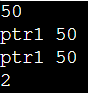##### weak_ptr:

It is also the same as shared_ptr. Here also more than one pointer can point to a single object. But it will not maintain Ref_count. So that’s why it is known as weak_ptr. So, the pointer will not have a strong hold on the object. The reason is if suppose the pointers are holding the object and requesting other objects, they may form a deadlock between the pointers. So, to avoid deadlock weak_ptr is useful. So, it doesn’t have Ref_count so it is more like unique_ptr but it allows the pointer to share an object, so it is more like shared_ptr. It is in between unique and shared which is not strict. It doesn’t bother how many pointers are pointing at an object.

In the next article, I am going to discuss InClass Initializer and Delegation of Constructors in C++ with Examples. Here, in this article, I try to explain Smart Pointers in C++ with Examples and I hope you enjoy this article. I would like to have your feedback. Please post your feedback, question, or comments about Smart Pointers in C++ with the Examples article.